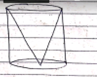From a solid right circular cylinder of a height 2.4cm and radius of 0.7cm,a right circular cone of same height and same radius is cut. Find the total surface area of the remaining solid. -CBSE  Class  -10 -Mathematics -Trigonometric -Trigonometric Subtopic –

# From a solid right circular cylinder of a height 2.4cm and radius of 0.7cm,a right circular cone of same height and same radius is cut. Find the total surface area of the remaining solid.-CBSE  Class  -10 -Mathematics -Trigonometric -Trigonometric Subtopic -

1. A
17.52cm2
2. B
17.57 cm2
3. C
17.11 cm2
4. D
18.57 cm2

Fill Out the Form for Expert Academic Guidance!l

+91

Live ClassesBooksTest SeriesSelf Learning

Verify OTP Code (required)

### Solution:

Height of cone & cylinder ,h =2.4 cm
Radius of cone and cylinder , r= 0.7 cm
Total surface area of remaining solid = curved surface area of cylinder +area of base +curved surface  area of cone  Total surface area of remaining  Solid = 2πrh +πr2+πrl
= 2*22/7(0.7)*2.4+22/7*(0.7)2+
= 22/7*(0.7)*√(0.7)2+ (2.4)2 =10.55 cm2 +1053 cm 2+ 22/7*0.7*2.5
=10.55 cm2 + 1.53 cm2+ 5.49 cm2 T..a of remaining solid = 17.57 cm2 Thus option (b) is correct .

## Related content

 Area of Square Area of Isosceles Triangle Pythagoras Theorem Triangle Formula Perimeter of Triangle Formula Area Formulae Volume of Cone Formula Matrices and Determinants_mathematics Critical Points Solved Examples Type of relations_mathematics+91

Live ClassesBooksTest SeriesSelf Learning

Verify OTP Code (required)